Skip to main content Skip to Search Box

Definition: right-angled triangle from The Penguin Dictionary of Science

A ➤triangle with one interior angle equal to a right angle.

Summary Article: Lesson 10-4: Right Triangles and the Pythagorean Theorem
from Homework Helpers: Basic Math and Pre-Algebra

A right triangle is a triangle with one angle measuring 90°. The right angle can be marked with a little square to make it stand out (as in Figure 10.10 on page 202). The side opposite the right angle is called the hypotenuse, and the other two sides are called the legs. The hypotenuse will always be longer than either of the two sides.

The Pythagorean Theorem describes the relationship between the length of the hypotenuse and the lengths of the two legs. The Pythagorean Theorem states that the sum of the squares of the lengths of the legs of a right triangle will equal the square of the length of the hypotenuse. This theorem may be easier to understand if we write it algebraically. Consider the right triangle in Figure 10.8. If a and b represent the lengths of the legs of a right triangle, and if c represents the length of the hypotenuse, the Pythagorean Theorem states that: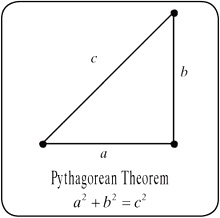Figure 10.8.

Many mathematicians have constructed proofs of the Pythagorean Theorem. Even non-mathematicians have discovered new ways to prove this theorem. In fact, President Garfield published a proof of the Pythagorean Theorem back in 1876.

One of the nice things about the Pythagorean Theorem is that it works both ways:

• Given any right triangle, the lengths of the three sides satisfy the relationship a2 + b2 = c2

• If the lengths of the three sides of a triangle satisfy the equation a2 + b2 = c2, where a and b are the lengths of the shorter sides and c is the length of the longest side, then the triangle is a right triangle.

The Pythagorean Theorem involves squaring lengths and solving for lengths by taking the square root of a number. Remember that if a number is a perfect square, its square root will be an integer. Otherwise, its square root will be an irrational number. We can use a calculator to approximate the square root of a number that is not a perfect square and round to get estimates for these irrational numbers.

Example 1

If the lengths of the two legs of a right triangle are 5 inches and 12 inches, find the length of the hypotenuse.

Solution: Let c represent the length of the hypotenuse and use the Pythagorean Theorem to find c.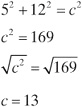So, the length of the hypotenuse is 13 inches.

Example 2

A right triangle has one leg that is three times longer than the other leg. If the length of the hypotenuse is 10 inches, find the lengths of the two legs.

Solution: Let a represent the length of the shorter leg. Then the length of the other leg is 3a. Use the Pythagorean Theorem to find a: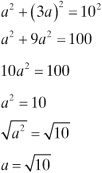So the length of the shorter leg is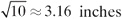, and the length of the longer leg is three times that, or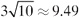. I used a calculator to approximate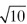and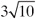, which are irrational numbers, and then rounded the answer.

Example 3

Sarah wants to measure the size of an angle to see if it is a right angle. She creates a triangle around it, as shown in Figure 10.9. Is Sarah's angle a right angle?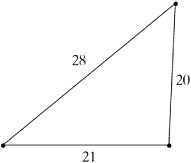Figure 10.9.

Solution: Check to see if the Pythagorean Theorem is satisfied. First, calculate the sum of the squares of the lengths of the two shorter sides:

202 + 212 = 400 + 441 = 841

Compare that result to the square of the length of the longer side:

282 = 784

Because the two results are not equal, the Pythagorean Theorem does not hold, so the triangle Sarah created is not a right triangle. Therefore, Sarah's angle is not a right angle.

Lesson 10-4 Review

Solve the following problems.

1. A right triangle has legs with length 4 and 6 inches. Find the perimeter of the triangle.

2. A right triangle has a hypotenuse that is twice as long as one of its legs. If the other leg is 6 inches long, find the perimeter of the triangle.

3. Is a triangle with sides of length 4, 7, and 10 centimeters a right triangle?Copyright 2011 © by Denise Szecsei

### Related Articles

##### Full text Article right triangle
The Penguin English Dictionary

noun NAmer a right-angled triangle. ...

##### Full text Article right triangle
Merriam-Webster's Collegiate(R) Dictionary

(1675) : a triangle having a right angle see triangle illustration

##### Full text Article right-angled triangle
The Penguin Dictionary of Mathematics

A triangle that has one interior angle equal to 90°. See Pythagoras’ theorem . ...

See more from Credo